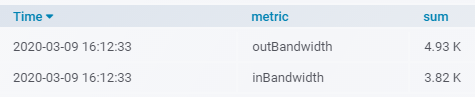# Divide two metric values with influxdb and grafana and show a single value as result for alerting

HI,

I have not used influxdb queries very often, so consider this question as a newbie question.

I want to divide inBandwidth with outBandwidth from the iftraffic check.

Example Query:

`SELECT sum("value") FROM "autogen"."iftraffic64_alias" WHERE ("hostname" =~ /myHost/ AND "service" =~ /Uplink ISP/ AND "metric" =~ /Bandwidth/) AND \$timeFilter GROUP BY "metric"`

gives:What i want is something like:

`SELECT sum("inBandwidth")/sum("outBandwidth") FROM "autogen"."iftraffic64_alias" WHERE ("hostname" =~ /myHost/ AND "service" =~ /Uplink ISP/ AND "metric" =~ /Bandwidth/) AND \$timeFilter`

Or in plain dEnglish: I want one number as result for alerting, which shows the ratio from up and download for the last 30 Minutes. I have the two metrics in one series.

Thanks for support.
Rafael

Found it:

SELECT sum(inBandwidth) / sum(outBandwidth) AS Ratio
FROM
(
SELECT sum(value) as outBandwidth FROM iftraffic64_alias WHERE “metric” = ‘outBandwidth’ AND Time > now() -30m
)
,
(
SELECT sum(value) as inBandwidth FROM iftraffic64_alias WHERE “metric” = ‘inBandwidth’ AND Time > now() -30m
)
GROUP BY hostname

1 Like